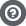Hello Guest

# [Problem] Ray-Line Selection With Triangles

• 1 Replies
• 4428 Views####Phyyl

•• 5##### [Problem] Ray-Line Selection With Triangles
« on: March 12, 2013, 00:23:55 »
Hi eveyone, I'm in great need of help here... I've been trying to make a selection system working with the camera and a normalized vector pointing in front of the camera... In other words, check if there's a collision with a triangle and a line. Unfortunatly, it doesn't work as good as I though and I'm having a hard time finding the errors/things I'm doing wrong!

What I'm seeing :

- It detects that I'm looking in the right direction, but only at a distance of approx. 1 from the plane.
- It only detects that I'm lloking in the right direction when I'm looking in between -45 and 45 degrees on the x axis (looking up/down)
- I never get the "collision" result...

Here's the bit of code where I check for a plane intersection with the line :
Code: [Select]
`public final class Plane { // o = origin (camera/eye position) // n = normalized vector for the direction the player is looking to // p = plane point (in this case the A point of a triangle) // pn = plane normalized vector public static Vector3f intersect(Vector3f o, Vector3f n, Vector3f p, Vector3f pn) { //tests if the line is perpendicular to the plane     if ((n.x * pn.x +     n.y * pn.y +     n.z * pn.z) != 0) {     // calculate t for the plane+line intersection     float t = (p.x*pn.x +        p.y*pn.y +        p.z*pn.z -        pn.x*pn.x -        pn.y*o.y -        pn.z*o.z) / (n.x*pn.x +         n.y*pn.y +         n.z*pn.z);     //tests if the line intersects IN FRONT of the player     if (t > 0)     //returns the position of the collision     return new Vector3f(o.x + t*n.x,     o.y + t*n.y,     o.x + t*n.z);     else     return null;     }     else {     return null;     }     }}`
... And here's the part where I check if the point is in the triangle :

Code: [Select]
`public class Triangle { public Vector3f a, b, c, n; public Triangle(Vector3f a, Vector3f b, Vector3f c, Vector3f n) { this.a = a; this.b = b; this.c = c; this.n = n; } public boolean intersect(Vector3f p) {        if (SameSide(p, a, b, c) && SameSide(p, a, b, c) && SameSide(p, a, b, c)) {            Vector3f vc1 = new Vector3f();            Vector3f.cross(sub(a, B)/>, sub(a, c), vc1);            if (Math.abs(Vector3f.dot(sub(a, p), vc1)) <= .01f)                return true;        }        return false;    }    private boolean SameSide(Vector3f p1, Vector3f p2, Vector3f A, Vector3f B)/> {        Vector3f cp1 = new Vector3f();        Vector3f.cross(sub(B, A), sub(p1, A), cp1);        Vector3f cp2 = new Vector3f();        Vector3f.cross(sub(B, A), sub(p2, A), cp2);        return Vector3f.dot(cp1, cp2) >= 0;    }        private Vector3f sub(Vector3f a, Vector3f B)/> {    Vector3f result = new Vector3f();    Vector3f.sub(a, b, result);    return result;    }        public void draw() {        glColor3f(1, 1, 1);    glBegin(GL_TRIANGLES);        glNormal3f(n.x, n.y, n.z);    glVertex3f(a.x, a.y, a.z);    glVertex3f(b.x, b.y, b.z);    glVertex3f(c.x, c.y, c.z);        glEnd();    }}`

Thank you so much!####quew8

•• 569
• Because Square Eyes Look More Real##### Re: [Problem] Ray-Line Selection With Triangles
« Reply #1 on: March 12, 2013, 17:21:02 »
Quote
// calculate t for the plane+line intersection
float t = (p.x*pn.x +
p.y*pn.y +
p.z*pn.z -
pn.x*pn.x - //MISTAKE HERE
pn.y*o.y -
pn.z*o.z) / (n.x*pn.x +
n.y*pn.y +
n.z*pn.z);
On the 5th line (Where my helpful comment is), there is a mistake. You wrote "pn.x * pn.x", it should be "pn.x * o.x"
Also perhaps it's just you trying to find bugs, but you seem to be working out n dot pn twice. And your writing out the individual operations when you could just be using the dot method in Vector you use later on. Made it a bit of a pain to debug (probably for you too) and this is where you made the mistake. Methods exist for a reason. Encapsulation ftw.

Thanks for listening.